Geodesic dome

Discussion in '3D Design Requests' started by Jettuh, Apr 8, 2009.

1. Hi guys,

atm i'm recreating The Swan aka The Hatch - tv series "lost" - but i'm having a problem at this point!

I'm trying to create a wire Geodesic Dome like in the picture below:but i don't know how to,
i know how to build the dome:but on this way: GeoSphere -> Icosa -> Hemisphere ticked on -> chamfer the lines so you get width, and then exture those lines for the thickness, and then remove the triangles.
problem now is, that i haven't got an inside and so on, i can't upload it (isn't watertight)Can anybody help me making a wired (sort of) geodesic dome?

I'm totally LOST2. That's the way I've created it: A sphere in Blender, exported to STL, imported with ViaCAD, change object type to solid, boolean operation for substracting the lower half with a cube, change object type to curves, used the tube function on all curves, exported to STL and automatic fixing the normales and manifolds with MeshLab filters. Finally re-imported in Blender and raytraced it:Would be better to create mathematically perfect without all the steps, with just one Blender script. I'll try this when I have more time. This is the file:

http://www.frank-buss.de/shapeways/dome.stl

Shapeways says it is printable:

http://www.shapeways.com/model/25509/geodesic_dome.html

There is no price tag, wouldn't make sense because of the provided source fileLast edited: Apr 8, 2009
3. Haha Jettuh,

Are you starting your own Lost museum?

I bet a lot of Losties out there envy you :smug:

Cheers,

Ralph

4. Hi Ralph,,ingame in the game Crysis:to bad it will be way to expensive to print it out,
yeah maybe it will be cheap when i create the whole hatch within 10cm by 10cm by 2cm high@frank,

to bad i haven't got both programs!
is there a way you know of, creating it in 3ds max?

Last edited: Apr 30, 2009
5. You could import the STL file. With HyperFun you can create it by yourself. Here is a little tutorial how to use it:

First install the polygonizer tool. Then create a text file dome.hf with this content:

Code:
```my_model(x, a)
{
-- array declarations
array center;
array rotated;

-- constants
pi = 3.14159;

-- create a cylinder
center = [0, 0, 0];
cylinder1 = hfCylinderX(x, center, 2);

-- create a rotated coordinate system
rotated = x;
rotated = x;
rotated = x;
angle = 32;
dummy = hfRotate3DZ(rotated, deg2rad * angle);

-- another cylinder, now with the rotated coordinates
cylinder2 = hfCylinderX(rotated, center, 2);

-- return constructive solid union of both cylinder
my_model = cylinder1 | cylinder2;
}
```
Then open the command prompt and call the hfp program like this:

Code:
```hfp dome.hf -w 400 -b 10
```
and you'll see a window where you can rotate and zoom (left and right mouse buttons while moving) the 3D model, which looks like this: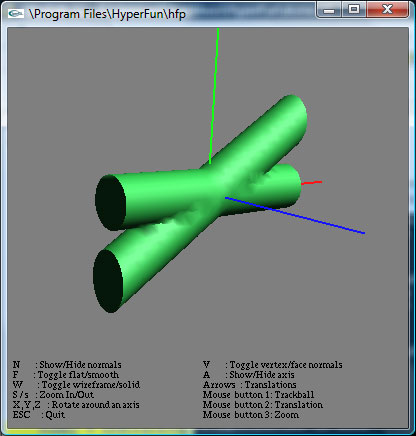The language is some kind of very restricted Basic, like Visual Basic 6 without returns and other limitations, e.g. you can't ignore return values, there are only floats and arrays of floats, assigning and initializing values is a pain if you know C++ or Python and the only control structures are loops and ifs, but at least this is sufficient for creating some nice models.

The concept of HyperFun is to call a function, my_model in the example, which is the default for the polygonizer tool, for all (x, y, z) points in the bounding box (specified by the "-b" parameter, which is interpreted as (-10,-10,-10)-(10,10,10), if you specify only "-b 10"). If the function returns a value >0, the point is inside the object, if it is 0, the point is on the surface and if it is < 0, the point is outside of the object. The x, y and z coordinates are provided with the x-array.

This is the same in 3D like I've implemented some time ago in 2D (see this Lisp sample) and sometimes it is called functional geometry. This is unusual at the beginning, because you don't describe an object, but you write a function and this function is called from the framwork with lots of points to produce the geometry. You have to think backward, like with function composition in mathematics or matrix multiplication (as you see in the example: for a rotated cylinder you don't create a cylinder and then rotate it, but you rotate the world and then return the points of the cylinder for this rotated coordinate system), but it is a very powerful concept. Sometimes this is reminiscent of functional programming like used in Haskell.

The line "cylinder1 = hfCylinderX(x, center, 2);" uses a predefined function (see this page for a reference of all available built-in functions), which returns a value <0, 0 or >0 for a given point. hfRotate3DZ rotates a vector about the z-axis. Finally the function returns the value of the union of both cylinders with the line "my_model = cylinder1 | cylinder2;".

But the cylinders are infinite long, so we need to intersect it with a cube. You can define your own functions, which can be called from the start function and with the a-parameter you can pass additional parameters. So it is possible to write a general purpose line function and draw a wireframe cube. For nice joints we add a sphere at the ends of the line. There are no functions with arbitrary arguments and no global variables, so it looks a bit obfuscates and redundant:

Code:
```line(x, a)
{
-- array declarations
array p;
array rotated;

-- constants
pi = 3.14159;
diameter = 2;

-- extract line start and end coordinates
xs = a;
ys = a;
zs = a;
xe = a;
ye = a;
ze = a;

-- extract current point
xc = x - xs;
yc = x - ys;
zc = x - zs;

-- line length
length = sqrt((xe-xs)^2+(ye-ys)^2+(ze-zs)^2);

-- distance from origin
length0 = sqrt(xs^2+ys^2+zs^2);

-- the following code is based on an article by Stan Melax in Game Programming Gems,
-- first calculate a quarternion, which represents the rotation between v0 and v1

-- the target vector
v0x = xe - xs;
v0y = ye - ys;
v0z = ze - zs;

-- normalize target vector
v0x = v0x / length;
v0y = v0y / length;
v0z = v0z / length;

-- the normalized cylinder vector
v1x = 1;
v1y = 0;
v1z = 0;

-- cross product of v0 and v1
cx = v0y*v1z - v0z*v1y;
cy = v0z*v1x - v0x*v1z;
cz = v0x*v1y - v0y*v1x;

-- dot product of v0 and v1
d = v0x * v1x + v0y * v1y + v0z * v1z;

-- special case: if the dot product is near -1, v0 and v1 are pointing in opposite directions and we can simply inverse the x-axis
if (d < 1e-5 - 1) then
xc = -xc;
else
-- calculate quarternion
sc = sqrt((1+d) * 2);
qx = cx / sc;
qy = cy / sc;
qz = cz / sc;
qw = sc / 2;

-- rotate coordinate system with quaternion
rw = -qx*xc-qy*yc-qz*zc;
rx = qw*xc+qy*zc-qz*yc;
ry = qw*yc+qz*xc-qx*zc;
rz = qw*zc+qx*yc-qy*xc;
xc = -rw*qx+rx*qw-ry*qz+rz*qy;
yc = -rw*qy+ry*qw-rz*qx+rx*qz;
zc = -rw*qz+rz*qw-rx*qy+ry*qx;
endif;

-- initialize rotated vector, translated by start point
rotated = xc;
rotated = yc;
rotated = zc;

-- create a cylinder
p = [0, 0, 0];

-- intersect with a cube
result = result & hfBlock(rotated, p, length, diameter, diameter);

-- add spheres at both line ends
p = [0, 0, 0];
result = result | hfSphere(rotated, p, radius);
p = length;
result = result | hfSphere(rotated, p, radius);

-- return result
line = result;
}

my_model(x, a)
{
-- array declarations
array coords;

result = -1;

-- pyramid base
coords = [ -5, -5,  0,    5, -5,  0 ]; result = result | line(x, coords);
coords = [  5, -5,  0,    5,  5,  0 ]; result = result | line(x, coords);
coords = [  5,  5,  0,   -5,  5,  0 ]; result = result | line(x, coords);
coords = [ -5,  5,  0,   -5, -5,  0 ]; result = result | line(x, coords);

-- sides
coords = [ -5, -5,  0,    0,  0,  7 ]; result = result | line(x, coords);
coords = [  5, -5,  0,    0,  0,  7 ]; result = result | line(x, coords);
coords = [  5,  5,  0,    0,  0,  7 ]; result = result | line(x, coords);
coords = [ -5,  5,  0,    0,  0,  7 ]; result = result | line(x, coords);

my_model = result;
}
```
This needs more sampling points, so render it with the additonal "-g" parameter:

Code:
```hfp dome.hf -w 400 -b 10 -g 80
```
The result: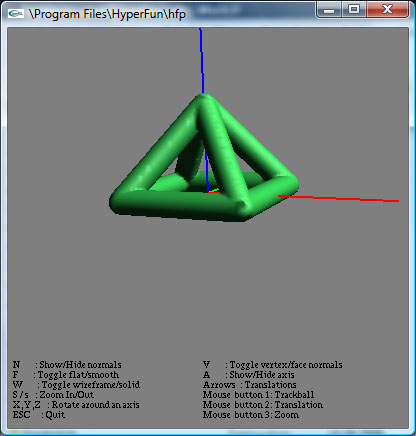Finally we can create the dome. The algorithm is based on this article. But the code needs ages to compute the polygones and then it is still not very detailed. And the code looks very ugly in the HyperFun language. This is not the fault of functional programming, e.g. in this example you can see a nice Haskell program written by me to draw some functional graphics.

Ok, back to more imperative languages. Now the same with Python in Blender. With a little framework I can write this:

Code:
```# create platonic solids
coords, faces = cubeData()
coords = translateArray(coords, [ -5, 0, 0 ])
coords, faces = octahedronData()
coords = translateArray(coords, [ 0, 0, 0 ])
coords, faces = dodecahedronData()
coords = translateArray(coords, [ 5, 0, 0 ])
coords, faces = icosahedronData()
coords = translateArray(coords, [ 0, 5, 0 ])
coords, faces = tetrahedronData()
coords = translateArray(coords, [ 5, 5, 0 ])
```
and I'll get this objects: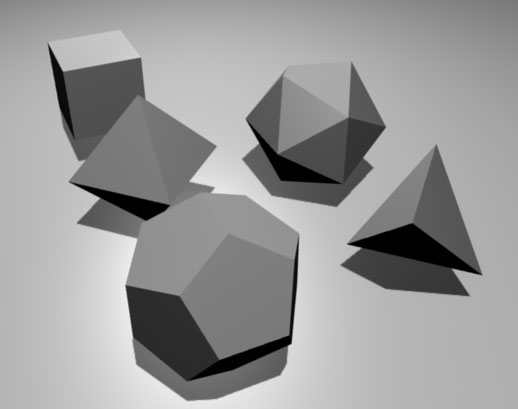The wireframe is just some cylinders and spheres for the joints: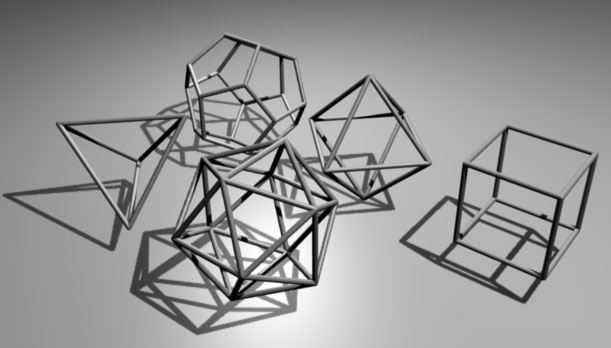The geodesic dome is an icosphere, where half of the sphere is deleted. This can be coded with my framework like this:

Code:
```domeRadius = 7
domeSubdivisions = 2
sphereSubdivisions = 2
steps = 50
coords, faces = half(coords, faces, -domeRadius / 10)
```
The result: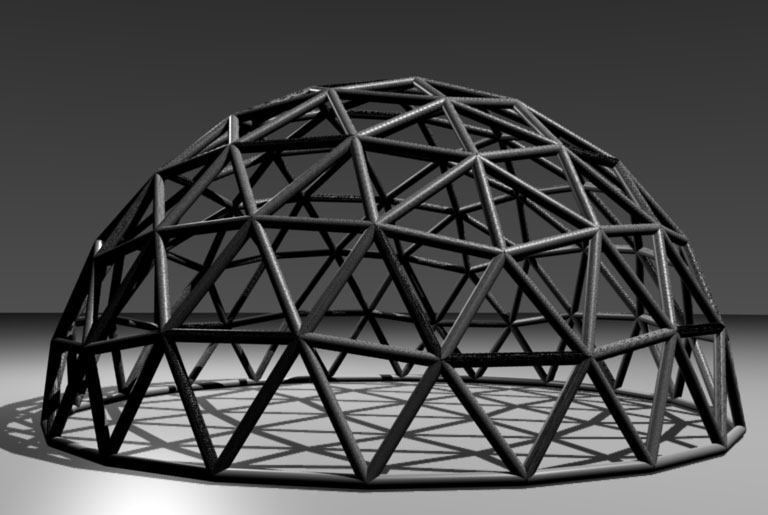I didn't use classes and operator overloading, so it should be very easy to port it to any other 3D scripting language, e.g. a 3ds script.

Here is the full source code, including the general framework functions. As you can see, it is much easier to implement this in Python than with HyperFun. Note: The rendering works, but there are still some problems with normals and manifolds, when I try to upload it to Shapeways, I'll analyze it.

6. Wow Jettuh, the hatch!

You never seize to amaze me :nod: . From now on, i will be following your work more closely, cause you're my new favourite modellerCheeers,

Ralph

7. well thank youat this moment i'm watching Lost all over again (don't ask me why,, probably because to watch the new episode of season 5,, you have to wait 7days,, have to do something between those days)

For the hatch, i would love to create it so it can be printed here.
But, i don't really know what to build of it,, like should i build the whole hatch,, or just build the hatch with just the walls so you can look into it..
(how much will it cost to produce it 1:1)

i was just watching season 3,, maybe tonight i'll create the cages..

The thing is, the more i build of the Dharma Initiatve,, the more i have to choose from like "what is the best thing to print"

Ohh one more thing,, when i was just writing about the cages,, why not the "barracks",, you know,, build the houses,, create those beautifull DHARMA VAN's

8. Yeah built it 1:1 :laughing:

What about the radio tower? Or the plane, or...the camp where the others live...or the underwater station, or the Black Rock?

Hmmzzz.... i can image you have a hard time choosingGood luck with it!

Last edited: May 4, 2009
9. yeah i was thinking about the place where the other live as well (the barracks)

but i still think i'm going to build the Swan,,
but then without a roof..
have to work in 15minutes,, so atm i'm eating.
i'm still thinking which scale i'm going to build it.
i think the best size is to make the walls wich are 2m about 4cm high!?

but then again, if i don't make a roof,, you miss a lot of nice details which are in the roof (like the living room,, has a beautifull roof) but if i do make the roof,, you hardly see anything from the insidebut the plane would be cool too,, except,, i think it would be hard to modelso much choiceswell time for me to go to work now,, i'll think about it over there.
won't be that busy on work tonight,, so i'll make some sketches and thoughts about the swan10. Jettuh: maybe try whilte detail, then you can create very small, but still detailed models.

BTW: I've updated the script at http://www.frank-buss.de/shapeways/dome.py . There were some problems with normales and duplicated surfaces, but now the result looks good. Still not printable, but stlfix.com fixed my models for free and I was able to add it to my designs.

The STL files:
http://www.frank-buss.de/shapeways/platonic.stl
http://www.frank-buss.de/shapeways/platonic-wireframe.stl
http://www.frank-buss.de/shapeways/dome.stl

You can buy it in my shop, it's only a small markuphttp://www.shapeways.com/model/28981/geodesic_dome.html
http://www.shapeways.com/model/28980/platonic_wireframe.html
http://www.shapeways.com/model/28414/platonic_solids.html

11. Hi frank,

thank you for helping me!
atm i'm trying to build the swan (the hatch i'm talking about in this topic) before i start creating the geodesic dome.
problem is that the walls would be 2,5cm high,, and i just got 1/10 of all the walls,and when checked with the price checker,, it was allready \$22so if that's allready 22dollars,,,i'm going to re think how to build it!?

best thing to do is make the easy walls with wood,, and the difficult walls printed!

thanx agaain12. Looks like your model has some thick walls and other things with high volume. You can try to make it hollow.

You can even make it hollow without any holes in it. E.g. this cube is a 10mm^3 cube, but with a 9mm^3 cube inside with flipped normals, which makes it hollow:

http://www.shapeways.com/model/29121/hollow_test.html

so it has a volume of 271mm^3 instead of 1000mm^3 and costs only \$0.54. If the walls are not smaller than 1mm it should be stable. And it is printable, but lots of powder would be locked inside, so I don't know, if Shapeway likes such models13. hi there,

well it wasn'ta thickness problem, but just the size..
the lenght is very long of the walls i made,, and the wallthickness is just 1,5mm.

i'll show a screenshot later today!